# Iodometry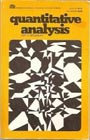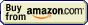## Potentiometric titration » Equivalence point calculation

Remember, that what we calculate is not the end point - but equivalence point.

To calculate potential at the equivalence point in redox titration, we can use exactly the same approximation we can use in every other titration type - that reaction proceeded almost to the end, so concentrations of products are given by the reaction stoichiometry. However, before solving an example in which we will use this approach, let's try to derive more or less general formula for equivalence point potential

Let's assume the reaction that takes place is

mAARed + mBBOx = mAAOx + mBBRed

where Red and Ox indices denote reduced and oxidized forms. We don't care about charges of these substances, we just assume that the reaction is properly balanced and that numbers of electrons exchanged in half reactions are nA and nB. Note, that this reaction equation is much more general than it may seem - while in many cases there are additional ions and molecules consumed/created, as long as their concentrations can be considered constant (either because they are in huge excess like water, or because they are buffered - like pH) they will simply became part of the formal potential of the half reaction.

For such a reaction, and for equivalence point, we can write two Nernst equations, describing potential of the solution: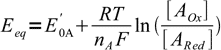1

and2

where E'0A and E'0B are formal potentials of both redox systems present.

These equations can be rewritten as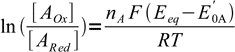3

and4

At equivalence point we have mixed stoichiometric amount of ARed and BOx. From the stoichiometry of the reaction we know that5

and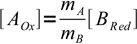6

Dividing equations side by side we get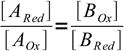7

That is in turn equivalent to8

(note sign change, this was done to switch nominator and denominator of the left hand side of the equation).

We are getting close to the final formula. Let's combine above equations: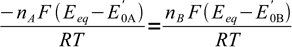9

canceling out R, T and F: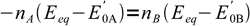10

and finally11

Note, that this equation, although often cited in analytical chemistry books, is not universal. It will work for all reactions that can be described by our skeletal equation. So for example it will work for titration described by the reaction

Fe2+ + Ce4+ → Fe3+ + Ce3+

or even

5Fe2+ + MnO4- + 8H+ → 5Fe3+ + Mn2+ + 4H2O

but not for

Cr2O72- + 6Fe3+ + 14H+ → 6Fe2+ + 2Cr3+ + 7H2O

as in this last reaction stoichiometry coefficient of the Cr2O72-/Cr3+ redox system changes from 1 on the left side of the equation to 2 on the right.

Calculate equivalence point potential if 0.02 M Fe(CN)64- is titrated with 0.1 M Ce4+. E0Ce4+/Ce3+ = 1.61 V, E0Fe(CN)63-/Fe(CN)64- = 0.36 V.

This is a straight application of the formula derived above. In both half reactions one electron is exchanged, so equivalence point potential is given by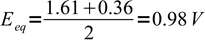12

Note, that we can ignore given concentrations of the titrant and titrated substance, as equivalence potential doesn't depend on them.

What is equivalence point potential when 0.1 M I2 solution is titrated with 0.1 M solution of S2O32-. E0I2/I- = 0.535 V, E0S4O62-/S2O32- = 0.08 V.

Reaction equation for this titration is

2S2O32- + I2 → S4O62- + 2I-

obviously we can't use our general formula (neither mA nor mB are constant). We have to use the general approach and assume that reaction went to (almost) completion.

We have to mix thiosulfate and iodine in 2:1 ratio. As solutions concentrations are identical that means we will use 2 volumes of thiosulfate and 1 volume of iodine, for the final volume of 3. Thus, taking both stoichiometry and dilution into account, we can write: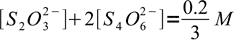13

and14

However, reaction is shifted so far to the right, that concentrations of bot reactants are (in the mass balance) negligible: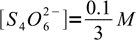15

and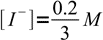16

We also know from the reaction stoichiometry that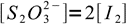17

Nernst equations for both systems are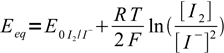1819

Let's combine Nernst equations with known information listed above, aiming at removing all variables but [I2]: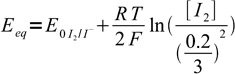20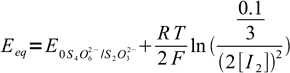21

We have two unknowns here. While we need Eeq, it is easier to calculate concentration of iodine first. Besides, that'll let us check if our assumption about the low iodine concentration was right. Subtracting second equation from the first one we get: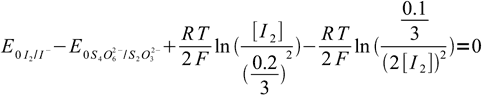22

After some rearranging and canceling: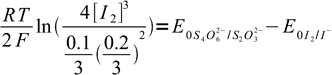23

or24

as iodide concentrations was 0.2/3 = 0.0667 our assumption that I2 concentration is much lower was correct. Entering known concentration into Nernst equation we get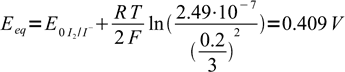25

and this is our equivalence potential. Note, that using derived formula we would get 0.308 V, almost 0.1 V off.